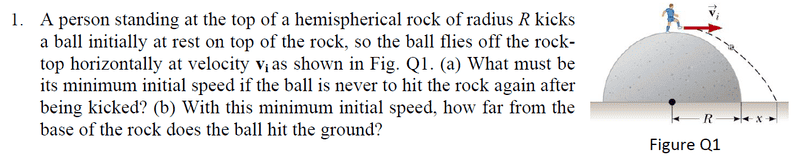# Projectile motion problem

## Homework StatementR = 1/2 gt^2
R+x = vt

## The Attempt at a Solution

For a), I tried to find t first, which is equal to √(2R/g). Then, I put t = √(2R/g) into the equation R+x = vt, and I found out that v = (R+x)/√(2R/g), is that correct?

For b), is the answer (R+x)?

hint: for the ball to reach the ground without hitting the rock, the condition is that the y-coordinate of ball should be greater than a certain height.

placing the origin at the center of sphere, ##R^2=x^2+y^2##
##(x,y)## are coordinates on the surface of hemisphere

use kinematic equation to determine the height of ball at each instant and replace ##t## from the equation.

taking ##x## as common coordinate, relate:

height ≥ ##y##

this is sufficient to solve the question. Try it.
P.S. My ##x## is different than the one asked!!!

tiny-tim
Homework Helper
hi david1111!For a), I tried to find t first, which is equal to √(2R/g). Then, I put t = √(2R/g) into the equation R+x = vt, and I found out that v = (R+x)/√(2R/g), is that correct?

For b), is the answer (R+x)?

yes, that's all correct, but you don't know what x is!

hint: what is the value of the centripetal acceleration?Centripetal acceleration= v^2 / R? But, how to relate it and x?

tiny-tim
Homework Helper
what is the value of it?

(you can find the value without knowing the speed)

haruspex